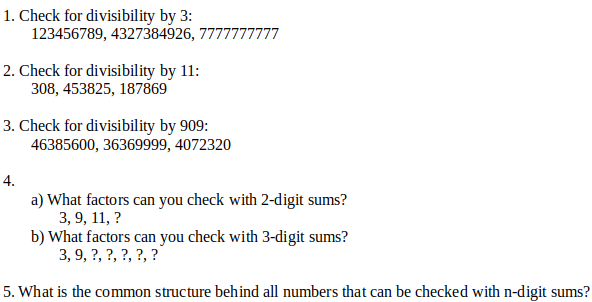# Math Contest #13 Results and Solution## Solution

The problem of this contest was a bit harder and I'm not overly surprised that nobody completely solved every task. I'll give here a short summary how you could have solved them:1. This can be done with the 1-digit sum:
123456789 → 1+2+3+4+5+6+7+8+9 = 45, 45 is divisible by 3 → yes
4327384926 → 48 → yes
7777777777 → 10*7 = 70 → no
2. For this you needed to apply the new concept of 2-digit sums:
308 → 3+08 = 11 → yes
453825 → 45+38+25 = 108 → no
187869 → 18+78+69 = 165 → yes
3. This was a bit more tricky, because you would need to either guess or find the concept asked in 5. to figure out which digit sum to use.
90911 = 9999 = 10⁴ → you needed to use the 4-digit sum:
46385600 → 4638+5600 = 10238 Here it is not so obvious, so I repeat the digit sum: 1+0238 = 239 → no
36369999 → 3636+9999 = 13635 I repeat it again: 1+3635 = 3636 = 4\*909 → yes
4072320 → 407+2320 = 2727 = 3\
909 → yes
4. a)
I thought this task would be the most easy.
You wouldn't even need to know anything about digit sums to solve this :P.
If you know a number is divisible by two factors which have no common prime factor, you also know that the number is also divisible by the product of those 2 factors:
3\11 = 33 and 9\11 = 99
Easy, right?
b)
This one could again only be solved with the knowledge of five.
You can check for all factors of 10³ - 1 = 999 with the 3-digit sum. Those factors are:
3, 9, 27, 37, 111, 333, 999
5. With any n-digit sum you can check for all factors of 10^n -1
Why is that?
You might need to figure it out yourself in a future contest. Until then I will keep it.

↓↑↓↑↓↑↓↑↓↑↓↑↓↑↓↑↓↑↓↑↓↑↓↑↓↑↓↑↓↑↓↑↓↑↓↑↓↑↓↑↓↑↓↑↓↑↓↑↓

### What is your chance of winning:

`p(You Win) = 1/n`, `n = number of entries`

↓↑↓↑↓↑↓↑↓↑↓↑↓↑↓↑↓↑↓↑↓↑↓↑↓↑↓↑↓↑↓↑↓↑↓↑↓↑↓↑↓↑↓↑↓↑↓↑↓

### List of participants with their entries:

Name solutions found comment
@tonimontana 2. 5. 10 STEM for you!
@mmunited 1. 2. 3. Although you didn't get the concept of 3., you got the right results → 7 STEM for you!
@golddeck 1. 2. (4.) You got many errors in 4., but you also got a few correct things. So I'll give 1.25 STEM for that task. → 4.25 STEM for you!

↓↑↓↑↓↑↓↑↓↑↓↑↓↑↓↑↓↑↓↑↓↑↓↑↓↑↓↑↓↑↓↑↓↑↓↑↓↑↓↑↓↑↓↑↓↑↓↑↓

## Winner draw:Congratulations @golddeck, you won 1 SBI!
↓↑↓↑↓↑↓↑↓↑↓↑↓↑↓↑↓↑↓↑↓↑↓↑↓↑↓↑↓↑↓↑↓↑↓↑↓↑↓↑↓↑↓↑↓↑↓↑↓